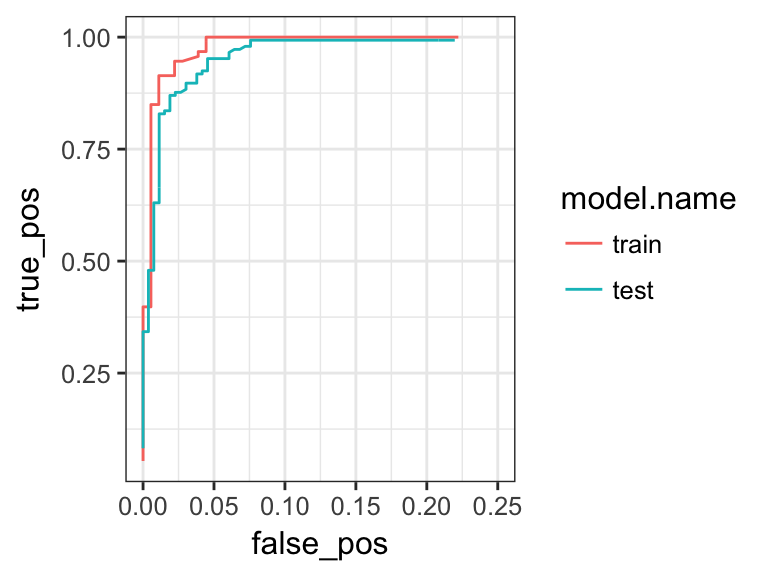## In-class worksheet 13

Feb 28, 2017

In this worksheet, we will use the libraries ggplot2, dplyr, and tidyr:

library(ggplot2)
theme_set(theme_bw(base_size=12)) # set default ggplot2 theme
library(dplyr)
library(tidyr)

# 1. Working with training and test data sets

We continue working with the biopsy data set:

biopsy <- read.csv("http://wilkelab.org/classes/SDS348/data_sets/biopsy.csv")

We will need this function from the last class, which calculates ROC curves:

calc_ROC <- function(probabilities, known_truth, model.name=NULL)
{
outcome <- as.numeric(factor(known_truth))-1
pos <- sum(outcome) # total known positives
neg <- sum(1-outcome) # total known negatives
pos_probs <- outcome*probabilities # probabilities for known positives
neg_probs <- (1-outcome)*probabilities # probabilities for known negatives
true_pos <- sapply(probabilities,
function(x) sum(pos_probs>=x)/pos) # true pos. rate
false_pos <- sapply(probabilities,
function(x) sum(neg_probs>=x)/neg)
if (is.null(model.name))
result <- data.frame(true_pos, false_pos)
else
result <- data.frame(true_pos, false_pos, model.name)
result %>% arrange(false_pos, true_pos)
}

The following code splits the biopsy data set into a random training and test set:

train_fraction <- 0.4 # fraction of data for training purposes
set.seed(126)  # set the seed to make your partition reproductible
train_size <- floor(train_fraction * nrow(biopsy)) # number of observations in training set
train_indices <- sample(1:nrow(biopsy), size = train_size)

train_data <- biopsy[train_indices, ] # get training data
test_data <- biopsy[-train_indices, ] # get test data

Fit a logistic regression model on the training data set, then predict the outcome on the test data set, and plot the resulting ROC curves. Limit the x-axis range from 0 to 0.25 to zoom into the ROC curve.

# model to use:
# outcome ~ clump_thickness + uniform_cell_size + uniform_cell_shape

glm.out.train <- glm(outcome ~ clump_thickness + uniform_cell_size + uniform_cell_shape,
data=train_data,
family=binomial)
test_pred <- predict(glm.out.train, test_data, type='response')
ROC.train <- calc_ROC(probabilities=glm.out.train$fitted.values, known_truth=train_data$outcome,
model.name="train")
ROC.test <- calc_ROC(probabilities=test_pred,
known_truth=test_data$outcome, model.name="test") ROCs <- rbind(ROC.train, ROC.test) ggplot(ROCs, aes(x=false_pos, y=true_pos, color=model.name)) + geom_line() + xlim(0, 0.25) ## Warning: Removed 347 rows containing missing values (geom_path).# 2. Area under the ROC curves The following code (commented out) calculates the area under multiple ROC curves: #ROCs %>% group_by(model.name) %>% # mutate(delta=false_pos-lag(false_pos)) %>% # summarize(AUC=sum(delta*true_pos, na.rm=T)) %>% # arrange(desc(AUC)) Use this code to calculate the area under the training and test curve for this following model. # model to use: # outcome ~ clump_thickness train_fraction <- 0.2 # fraction of data for training purposes set.seed(101) # set the seed to make your partition reproductible n_obs <- nrow(biopsy) # number of observations in biopsy data set train_size <- floor(train_fraction * nrow(biopsy)) # number of observations in training set train_indices <- sample(1:n_obs, size = train_size) train_data <- biopsy[train_indices, ] # get training data test_data <- biopsy[-train_indices, ] # get test data glm.out.train <- glm(outcome ~ clump_thickness, data=train_data, family=binomial) test_pred <- predict(glm.out.train, test_data, type='response') ROC.train <- calc_ROC(probabilities=glm.out.train$fitted.values,
known_truth=train_data$outcome, model.name="train") ROC.test <- calc_ROC(probabilities=test_pred, known_truth=test_data$outcome,
model.name="test")
ROCs <- rbind(ROC.train, ROC.test)
ROCs %>% group_by(model.name) %>%
mutate(delta=false_pos-lag(false_pos)) %>%
summarize(AUC=sum(delta*true_pos, na.rm=T)) %>%
arrange(desc(AUC))
## # A tibble: 2 × 2
##   model.name       AUC
##       <fctr>     <dbl>
## 1       test 0.9433136
## 2      train 0.9299145

# 3. If this was easy

Write code that generates an arbitrary number of random subdivisions of the data into training and test sets, fits a given model, calculates the area under the curve for each test data set, and then calculates the average and standard deviation of these values.

# function that does the heavy lifting
calc_AUC <- function(data, model, train_fraction)
{
n_obs <- nrow(data) # number of observations in data set
train_size <- floor(train_fraction * nrow(data)) # number of observations in training set
train_indices <- sample(1:n_obs, size = train_size)

train_data <- data[train_indices, ] # get training data
test_data <- data[-train_indices, ] # get test data
glm.out.train <- glm(model, data=train_data, family=binomial)
test_pred <- predict(glm.out.train, test_data, type='response')
ROC.train <- calc_ROC(probabilities=glm.out.train$fitted.values, known_truth=train_data$outcome,
model.name="training.AUC")
ROC.test <- calc_ROC(probabilities=test_pred,
known_truth=test_data\$outcome,
model.name="test.AUC")
ROCs <- rbind(ROC.train, ROC.test)
# calculate AUCs
ROCs %>% group_by(model.name) %>%
mutate(delta=false_pos-lag(false_pos)) %>%
summarize(AUC=sum(delta*true_pos, na.rm=T)) %>%
mutate(row=1) %>%
select(-row)
}

# function that does repeated random subsampling validation
subsample_validate <- function(data, model, train_fraction, replicates)
{
reps <- data.frame(rep=1:replicates) # dummy data frame to iterate over
reps %>% group_by(rep) %>% # iterate over all replicates
do(calc_AUC(data, model, train_fraction)) %>% # run calc_AUC for each replicate
ungroup() %>%     # ungroup so we can summarize
summarize(mean.train.AUC=mean(training.AUC),        # summarize
sd.train.AUC=sd(training.AUC),
mean.test.AUC=mean(test.AUC),
sd.test.AUC=sd(test.AUC)) %>%
mutate(train_fraction=train_fraction, replicates=replicates) # add columns containing meta data
}

Now that we have these two functions, we can use them to complete the exercise:

train_fraction <- 0.2 # fraction of data for training purposes
replicates <- 10 # how many times do we want to randomly sample
set.seed(116) # random seed
model <- outcome ~ clump_thickness + normal_nucleoli # the model we want to fit
subsample_validate(biopsy, model, train_fraction, replicates)
## # A tibble: 1 × 6
##   mean.train.AUC sd.train.AUC mean.test.AUC sd.test.AUC train_fraction
##            <dbl>        <dbl>         <dbl>       <dbl>          <dbl>
## 1      0.9703497   0.01179102     0.9727011 0.002249264            0.2
## # ... with 1 more variables: replicates <dbl>
# redo with a different model
model2 <- outcome ~ clump_thickness + normal_nucleoli + marg_adhesion
subsample_validate(biopsy, model2, train_fraction, replicates)
## # A tibble: 1 × 6
##   mean.train.AUC sd.train.AUC mean.test.AUC sd.test.AUC train_fraction
##            <dbl>        <dbl>         <dbl>       <dbl>          <dbl>
## 1      0.9848656  0.008365815     0.9842488 0.003611386            0.2
## # ... with 1 more variables: replicates <dbl>